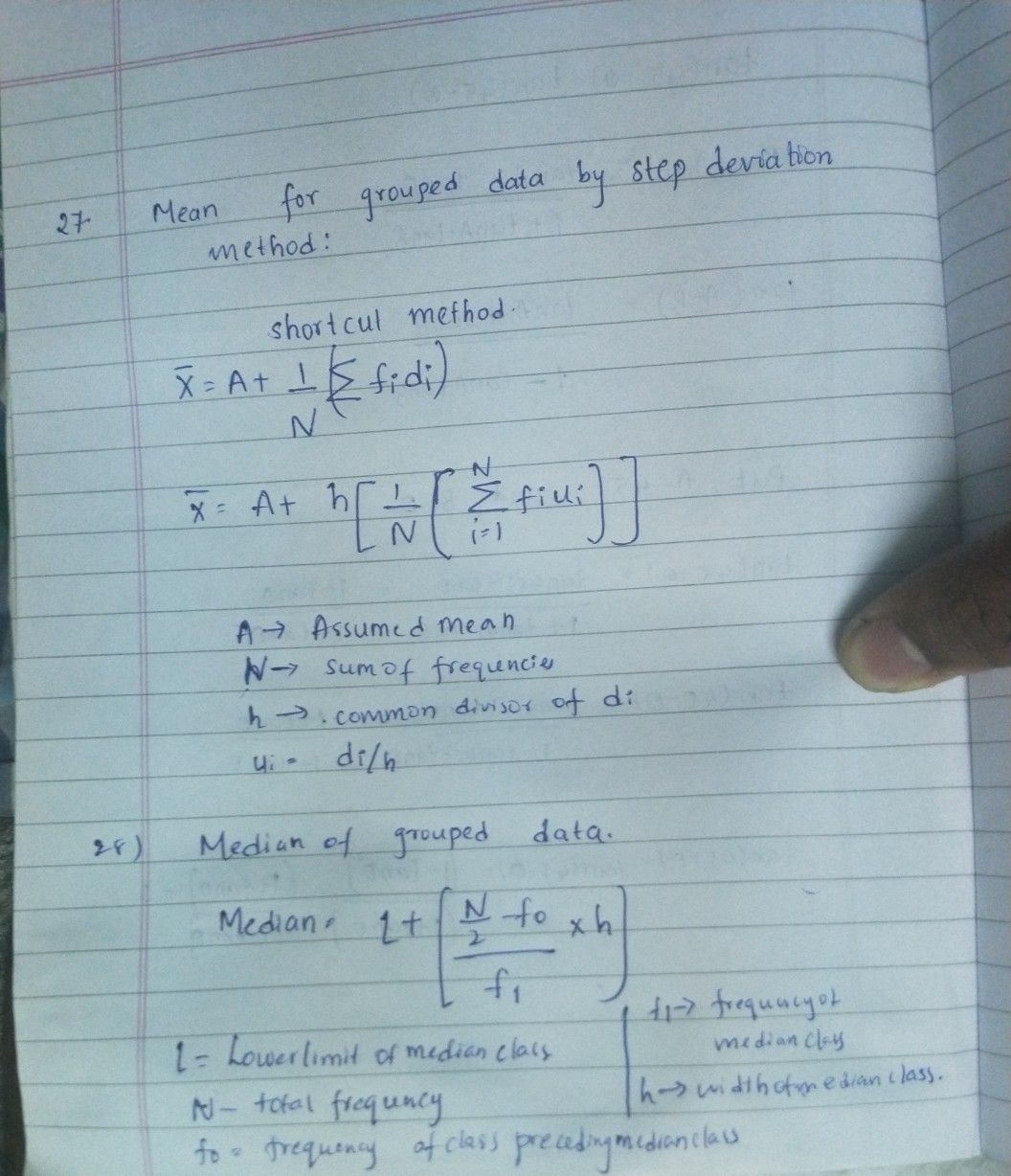Symbol
Problem$No$ of families $7$ $8$ $2$ $2$ $1$ 27.Write the formula for mean for grouped data by step deviation method and $explain$ each term in it? $28$ Write the formula for median for grouped data ? Explain each term in it.?
10th-13th grade
Other
Search count: 107
SolutionQanda teacher - saishankarthank you for using qanda
your feedback is valuable
please rate my answer